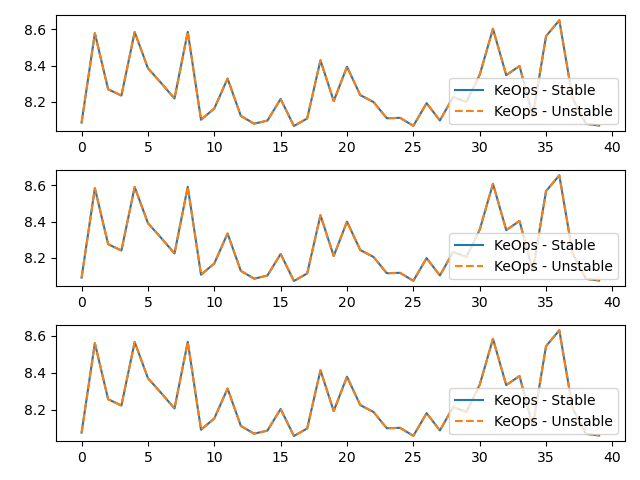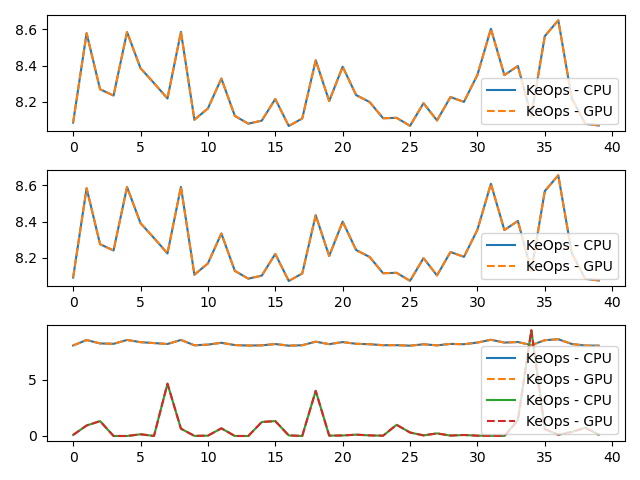# Vectorial LogSumExp reduction¶

Let’s compute the (3000,1) tensor $$c$$ whose entries $$c_i$$ are given by:

$c_i = \log \left[ \sum_j \exp\left( (p-a_j)^2 \exp(x_i+y_j) \right) b_j\right]$

where

• $$x$$ is a (3000,1) tensor, with entries $$x_i$$.

• $$y$$ is a (5000,1) tensor, with entries $$y_j$$.

• $$a$$ is a (5000,1) tensor, with entries $$a_j$$.

• $$p$$ is a scalar, encoded as a vector of size (1,).

• $$b$$ is a (5000,3) tensor, with entries $$b_j$$.

## Setup¶

Standard imports:

import time

import matplotlib.pyplot as plt
import torch

from pykeops.torch import Genred


Declare random inputs:

M = 3000
N = 5000

dtype = 'float32' # Could be 'float32' or 'float64'
torchtype = torch.float32 if dtype == 'float32' else torch.float64

x = torch.rand(M, 1, dtype=torchtype)
y = torch.rand(N, 1, dtype=torchtype, requires_grad=True)
a = torch.rand(N, 1, dtype=torchtype)
p = torch.rand(1, dtype=torchtype)
b = torch.rand(N, 3, dtype=torchtype)


## Define a custom formula¶

formula = 'Square(p-a)*Exp(x+y)'
formula2 = 'b'
variables = ['x = Vi(1)',  # First arg   : i-variable, of size 1 (scalar)
'y = Vj(1)',  # Second arg  : j-variable, of size 1 (scalar)
'a = Vj(1)',  # Third arg   : j-variable, of size 1 (scalar)
'p = Pm(1)',  # Fourth arg  : Parameter,  of size 1 (scalar)
'b = Vj(3)']  # Fifth arg   : j-variable, of size 3 (vector)

start = time.time()


Our log-sum-exp reduction is performed over the index $$j$$, i.e. on the axis 1 of the kernel matrix. The output c is an $$x$$-variable indexed by $$i$$.

my_routine = Genred(formula, variables, reduction_op='LogSumExp', axis=1, dtype=dtype, formula2=formula2)
c = my_routine(x, y, a, p, b, backend='CPU')

# N.B.: By specifying backend='CPU', we can make sure that the result is computed using a simple C++ for loop.
print('Time to compute the convolution operation on the cpu: ', round(time.time()-start,5), 's', end=' ')


Out:

Time to compute the convolution operation on the cpu:  0.02336 s


We compare with the unstable, naive computation “Log of Sum of Exp”:

my_routine2 = Genred('Exp('+formula+')*'+formula2, variables, reduction_op='Sum', axis=1, dtype=dtype)
c2 = torch.log(my_routine2(x, y, a, p, b, backend='CPU'))
print('(relative error: ',((c2-c).norm()/c.norm()).item(), ')')

# Plot the results next to each other:
for i in range(3):
plt.subplot(3, 1, i+1)
plt.plot(c.detach().cpu().numpy()[:40, i], '-', label='KeOps - Stable')
plt.plot(c2.detach().cpu().numpy()[:40, i], '--', label='KeOps - Unstable')
plt.legend(loc='lower right')
plt.tight_layout() ; plt.show()Out:

(relative error:  1.7385443129569467e-07 )


Now, let’s compute the gradient of $$c$$ with respect to $$y$$. Since $$c$$ is not scalar valued, its “gradient” $$\partial c$$ should be understood as the adjoint of the differential operator, i.e. as the linear operator that:

• takes as input a new tensor $$e$$ with the shape of $$c$$

• outputs a tensor $$g$$ with the shape of $$y$$

such that for all variation $$\delta y$$ of $$y$$ we have:

$\langle \text{d} c . \delta y , e \rangle = \langle g , \delta y \rangle = \langle \delta y , \partial c . e \rangle$

Backpropagation is all about computing the tensor $$g=\partial c . e$$ efficiently, for arbitrary values of $$e$$:

# Declare a new tensor of shape (M,1) used as the input of the gradient operator.
# It can be understood as a "gradient with respect to the output c"
# and is thus called "grad_output" in the documentation of PyTorch.
e = torch.rand_like(c)
start = time.time()
# PyTorch remark : grad(c, y, e) alone outputs a length 1 tuple, hence the need for  at the end.

print('Time to compute gradient of convolution operation on the cpu: ', round(time.time()-start,5), 's', end=' ')


Out:

Time to compute gradient of convolution operation on the cpu:  0.02862 s


We compare with gradient of Log of Sum of Exp:

g2 = grad(c2, y, e)
print('(relative error: ',((g2-g).norm()/g.norm()).item(), ')')

# Plot the results next to each other:
plt.plot(g.detach().cpu().numpy()[:40], '-', label='KeOps - Stable')
plt.plot(g2.detach().cpu().numpy()[:40], '--', label='KeOps - Unstable')
plt.legend(loc='lower right')
plt.tight_layout() ; plt.show()Out:

(relative error:  1.813483834212093e-07 )


## Same operations performed on the Gpu¶

Of course, this will only work if you own a Gpu…

if torch.cuda.is_available():
# first transfer data on gpu
pc, ac, xc, yc, bc, ec = p.cuda(), a.cuda(), x.cuda(), y.cuda(), b.cuda(), e.cuda()
# then call the operations
start = time.time()
c3 = my_routine(xc, yc, ac, pc, bc, backend='GPU')
print('Time to compute convolution operation on the gpu:',round(time.time()-start,5), 's ', end='')
print('(relative error:', float(torch.abs((c2 - c3.cpu()) / c2).mean()), ')')
start = time.time()
print('Time to compute gradient of convolution operation on the gpu:', round(time.time()-start,5), 's ', end='')
print('(relative error:', float(torch.abs((g2 - g3.cpu()) / g2).mean()), ')')

# Plot the results next to each other:
for i in range(3):
plt.subplot(3, 1, i+1)
plt.plot(c.detach().cpu().numpy()[:40, i], '-', label='KeOps - CPU')
plt.plot(c3.detach().cpu().numpy()[:40, i], '--', label='KeOps - GPU')
plt.legend(loc='lower right')
plt.tight_layout() ; plt.show()

# Plot the results next to each other:
plt.plot(g.detach().cpu().numpy()[:40], '-', label='KeOps - CPU')
plt.plot(g3.detach().cpu().numpy()[:40], '--', label='KeOps - GPU')
plt.legend(loc='lower right')
plt.tight_layout() ; plt.show()Out:

Time to compute convolution operation on the gpu: 0.00198 s (relative error: 9.602010919707027e-08 )
Time to compute gradient of convolution operation on the gpu: 0.00486 s (relative error: 6.517857400467619e-07 )


Total running time of the script: ( 0 minutes 0.574 seconds)

Gallery generated by Sphinx-Gallery